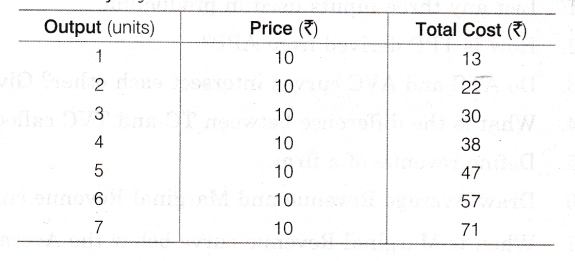# Given below is a cost and revenue schedule of a producer

Given below is a cost and revenue schedule of a producer. At what level of output is the producer in equilibrium? Give reasons for your answer.Producer is in equilibrium at 6th unit of output.
Reason At output level of 5th and 6th units, the difference between Total Revenue and Total Cost (i.e. profit) is maximum which is equal to 3 in both the cases. But, producer is in equilibrium at 6th unit only where MR= MC, i.e. Rs. 10 as MC>MR afterwards.